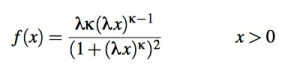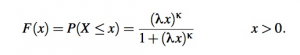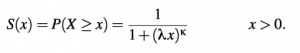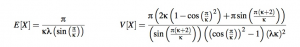# Fisk Distribution (Log-Logistic): Definition

Share on

The Fisk distribution, also called the log-logistic distribution, is a continuous probability distribution with many applications: from modeling the distribution of wealth or income in economics, to stream flow rates in hydrology to the lifetime of an organism in biostatistics. It is especially useful in modeling situations where the rate something is happening increases initially, and then after some time begins to decrease.

The term ‘fisk distribution’ is primarily used in economics; in other fields, ‘log-logistic distribution’ is more common.

The fisk distribution has two parameters, a scale parameter and a shape parameter. Both are positive numbers. To say that a random variable x has the log-logistic distribution, we write x ~ loglogistic(λ κ). Here λ is the scale parameter and κ is the shape parameter; both, again, are positive.

## The Fisk Distribution’s Probability Density Function

The probability distribution function (pdf) is given by:where λ is the scale parameter and κ is the shape parameter.

## Other Important Functions

The cumulative distribution function, on the support of X, is given by:The survival function, on the support of X, is given by:The population mean and variance, for a data set that follows the fisk distribution, is given by:The median of x is just 1/λ.

## References

Al-Shomrani, Shawky, Arif & Aslam. Log-logistic distribution for survival data analysis using MCMC. SpringerPlus (2016) 5:1774. DOI 10.1186/s40064-016-3476-7. Retrieved from https://link.springer.com/content/pdf/10.1186/s40064-016-3476-7.pdf on May 18, 2018

Leemis, Larry. Log Logistic Distribution. Retrieved form
http://www.math.wm.edu/~leemis/chart/UDR/PDFs/Loglogistic.pdf on May 18, 2018

World Heritage Encyclopedia, Fisk Distribution. Retrieved from http://self.gutenberg.org/articles/fisk_distribution on May 6, 2018

CITE THIS AS:
Stephanie Glen. "Fisk Distribution (Log-Logistic): Definition" From StatisticsHowTo.com: Elementary Statistics for the rest of us! https://www.statisticshowto.com/fisk-distribution/
---------------------------------------------------------------------------Need help with a homework or test question? With Chegg Study, you can get step-by-step solutions to your questions from an expert in the field. Your first 30 minutes with a Chegg tutor is free!